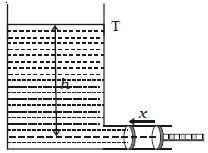# Total Energy of Liquid in Pressure Energy

A liquid in motion possesses pressure energy, kinetic energy and potential energy.

Pressure energy:Consider a liquid of density p contained in a wide tank T having a side tube near the bottom of the tank as shown in Figure. A friction less piston of cross sectional area ‘a’ is fitted to the side tube. Pressure exerted by the liquid on the piston is P = h ρ g.

Where h is the height of liquid column above the axis of the side tube. If x is the distance through which the piston is pushed inwards, then

Volume of liquid pushed into the tank = ax

Mass of the liquid pushed into the tank = axρ

As the tank is wide enough and a very small amount of liquid is pushed inside the tank, the height h and hence the pressure P may be considered as constant.

Work done in pushing the piston through the distance x = Force on the piston x distance moved

(i.e) W = Pax

This work done is the pressure energy of the liquid of mass axρ.

Pressure energy per unit mass of the liquid = Pax/asρ = P/ρ